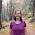## Wednesday, May 14, 2014

### Plotting bars at the tips of a circular tree

Today Matt Helmus posted the following request to the R-sig-phylo mailing list:

"Does anyone know of R code (or perhaps another program) to plot bars across the tips of a radial/fan phylogeny? Specifically, I have a large phylogeny and a corresponding vector of continuous trait values for the tips, and while I could use those values to vary the color and size of the tip labels, or also to plot points of varying color or size at those tips, I think a better depiction of the data would be to plot bars that vary in height."

Naturally, this appealed to me. The biggest challenge is resuscitating high-school (or perhaps elementary school) trig (think SOH-CAH-TOA) to get the position of the vertices for each tip bar/rectangle correct. Here is my attempt. Note that it doesn't contain much in the ways of bells-and-whistles, but these could easily be added:

plotFan.wBars<-function(tree,x,scale=1,width=NULL){
lims=c(-max(nodeHeights(tree))-scale*max(x),
max(nodeHeights(tree))+scale*max(x))
obj<-plotTree(tree,type="fan",ftype="off",
xlim=lims,ylim=lims)
if(is.null(width)) width<-(par()\$usr-par()\$usr)/100
w<-width
x<-x[tree\$tip.label]*scale
for(i in 1:length(x)){
dx<-obj\$xx[i]
dy<-obj\$yy[i]
theta<-atan(dy/dx)
x1<-dx+(w/2)*cos(pi/2-theta)
y1<-dy-(w/2)*sin(pi/2-theta)
x2<-dx-(w/2)*cos(pi/2-theta)
y2<-dy+(w/2)*sin(pi/2-theta)
s<-if(dx>0) 1 else -1
x3<-s*x[i]*cos(theta)+x2
y3<-s*x[i]*sin(theta)+y2
x4<-s*x[i]*cos(theta)+x1
y4<-s*x[i]*sin(theta)+y1
polygon(c(x1,x2,x3,x4),c(y1,y2,y3,y4),col="grey")
}
invisible(obj)
}

The two inobvious input arguments, scale & width, control the scale of the tip bars relative to the total height of the tree & the width of bars, respectively.

Let's try it:

> tree<-pbtree(n=50,scale=1)
> x<-fastBM(tree)
> x<-abs(x)
> plotFan.wBars(tree,x,scale=0.2)

That's all.

#### 1 comment:

1.This comment has been removed by the author.

Note: due to the very large amount of spam, all comments are now automatically submitted for moderation.